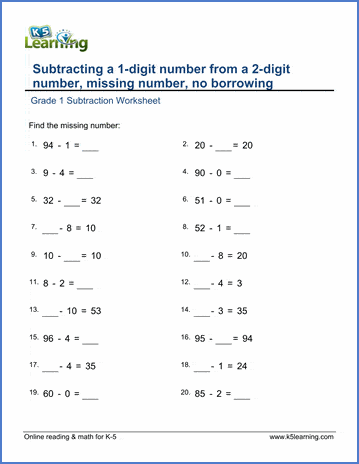# 1st Grade Math Worksheets: Subtraction

Our grade 1 subtraction worksheets provide practice in solving basic subtraction problems. Exercises begin with simple subtraction facts using pictures or number lines and progress to subtraction of 2-digit numbers in columns. Our grade 1 exercises do not require regrouping (or "borrowing").

## Introduction to subtraction

Subtract by crossing out objects

Subtract by drawing & crossing out

Solve subtraction word problems with objects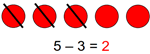Select the subtraction sentence

Write subtraction sentences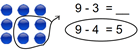Subtract using number lines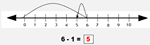## Mental subtraction

Subtract within 0-10 9 - 4 =
Subtract by counting back 9 - 4 =
Subtract by counting up 9 - 4 =

Missing minuend or subtrahend (within 10) 8 - __ = 2
Subtract within 1-20 17 - 3 =
Subtract 1-digit from 1-100 (no regrouping) 67 - 6 =
Subtract 1-digit from 1-100 (missing numbers) 67 - __ = 61

Subtract whole tens 70 - 40 =
Subtract whole tens (missing numbers) 70 - __ = 30
Subtract from whole tens 20 - 3 =

## Subtracting in columns

Subtract 1-digit from a 2-digit number (no borrowing) 67
- 3

Subtract 2-digit numbers (no borrowing) 74
- 21

Subtract in columns (missing numbers) 69
-
44

Fact families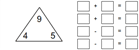Add & subtract 3 numbers 10 + 5 - 1 =
Add & subtract 4 numbers 6 + 7 - 1 - 7=

## Subtraction word problems

Subtract 1-digit numbers Word Problems
Subtract numbers < 50 Word Problems
Add / subtract word problems Word Problems
Add / subtract word problems (numbers < 50) Word Problems